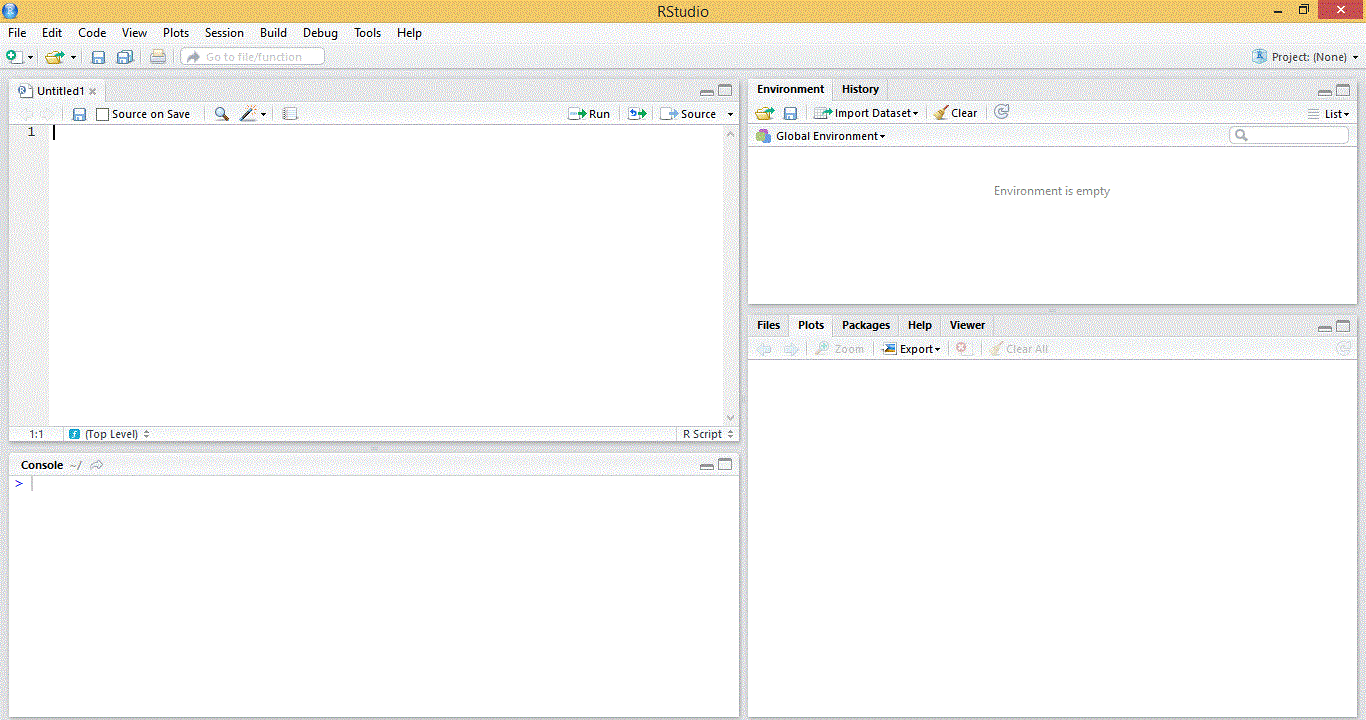Introduction to R Software

R is a open source statistical software. R software can be downloaded from the following link . https://www.r-project.org/
There are few GUI available for R software
Starting the R environment (This page uses the R-Studio GUI for examples )
Please double click the the R-Studio icon and the following window opensThe left top most window is called 'source' where the r codes are usually written and left bottom window is the R-Console
where the codes are executed. Press the run button in the source window to run the code

Basic Concepts

1. Package and Library
R software uses the concept called packages for various operations which needs to be downloaded and installed in the space
called R Library. To use the package, type the following syntax and press run
library

2. Frequently used packages

The following are the frequently used packages

 S.No Package Type remarks 1 stat Basic Statistical function Builtin 2 Randomforest Classification and regression 3 foreign Data Connection Read and write data from other packages such as SPSS and SAS 4 XL connect Data Connection Read and write data from excel 5 RODBC Data Connection Connect to a database through ODBC 6 xtable Data Handling 7 hmisc Data Handling 8 rcurl Data Handling 9 plyr Data Handling 10 pastecs Descriptive Statistics Display summary statistics 11 ggplot2 Graphical display Graphical display of data 12 lattice Graphical Display Graphical display of data 13 mlogit Logit Model Maximum Likelihood 14 nlme Mixed Effects Model Linear and Nonlinear 15 lme4 Mixed Effects Model Linear and Generalized S4 models 16 caret Predictive Modeling Predictive Modeling 17 xml Reading and creating xml and html files 18 Design Regression 19 Survey Survey 20 Survival Survival Analysis Survival Analysis 21 tm Text Mining Text Mining package

3. Data in R

Data is stored and represented as vector in R software. The function "c" can be used to store the data as vector.
For example if want to store the age of 5 patients
> age = c(50,45,30,41,24)          # R code

Here the age will be stored as vector age[].
>  5  # R output
Age of first patient can be obtained by specifying the vector element age.

We can also use the following function to store data
1. Data entry through key in
age = scan()

# R code 2. Entry through data editor
age = c(0)   # R code initial value of  age to be changed in the editor
Data.entry(age)  # R code

4. Basic Functions on Data

We can apply basic function on our data
>max(age)                  # R code maximum
>min(age)                  # R code minimum
>mean(age)               # R code mean
>sd(age)               # R code mean
>median(age)            # R code median
>table(age)              # R code frequency table
>summar(age)        # R code to list summary - mean,max,min,median,1st quartile, 3quartile